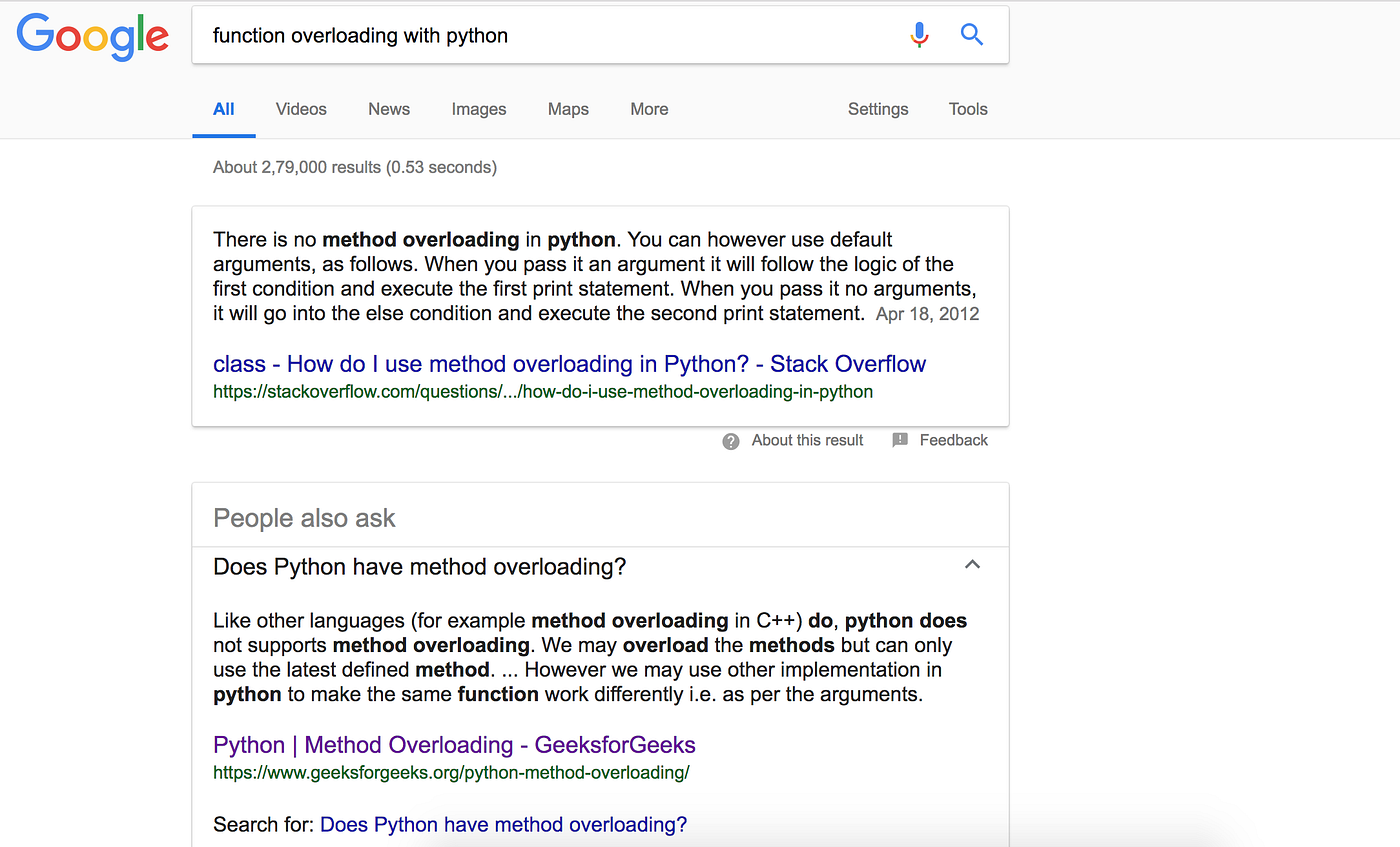# Second Chapter : De Mystifying Decorators in Python

`class ImportantStuff(object):    @time_this    def do_stuff_1(self):        ...    @time_this    def do_stuff_2(self):        ...    @time_this    def do_stuff_3(self):        ...`

# What is Bounded Knapsack ?

## Problem Statement #

Given two integer arrays to represent weights and profits of ’N’ items, we need to find a subset of these items which will give us maximum profit such that their cumulative weight is not more than a given number ‘C’. Each item can only be selected once, which means either we put an item in the knapsack or skip it.

# Polymorphism in Python`# to calculate area of rectangledef area(length, breadth):        calc = length * breadth    print calc#to…`